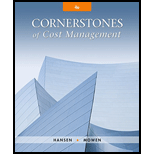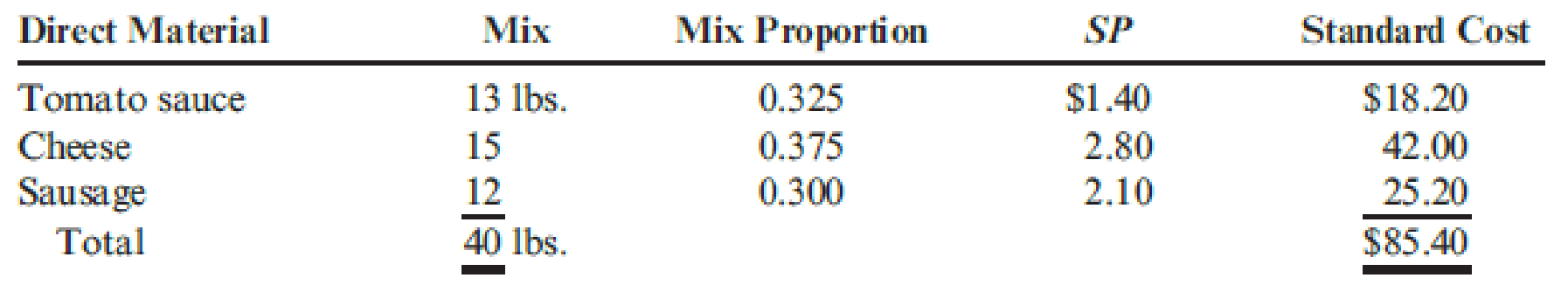# Mangia Pizza Company makes frozen pizzas that are sold through grocery stores. Mangia developed the following standard mix for spreading on premade pizza shells to produce 16 giant-size sausage pizzas. Mangia put a batch of 2,000 pounds of direct materials (enough for 800 frozen sausage pizzas) into process. Of the total, 700 pounds were tomato sauce, 840 pounds were cheese, and the remaining 460 pounds were sausage. The actual yield was 780 pizzas. Required: 1. Calculate the standard mix ( SM ) in pounds for tomato sauce, for cheese, and for sausage. 2. Calculate the mix variance. 3. Calculate the actual proportion used of tomato sauce, cheese, and sausage. Use these results to explain the direction (favorable or unfavorable) of the mix variance. 4. What if of the total 2,000 pounds of ingredients put into process, 700 pounds were tomato sauce, 700 pounds were cheese, and 600 pounds were sausage? How would that affect the mix variance?### Cornerstones of Cost Management (C...

4th Edition
Don R. Hansen + 1 other
Publisher: Cengage Learning
ISBN: 9781305970663

#### Solutions

Chapter
Section### Cornerstones of Cost Management (C...

4th Edition
Don R. Hansen + 1 other
Publisher: Cengage Learning
ISBN: 9781305970663
Chapter 9, Problem 9CE
Textbook Problem
194 views

## Mangia Pizza Company makes frozen pizzas that are sold through grocery stores. Mangia developed the following standard mix for spreading on premade pizza shells to produce 16 giant-size sausage pizzas.Mangia put a batch of 2,000 pounds of direct materials (enough for 800 frozen sausage pizzas) into process. Of the total, 700 pounds were tomato sauce, 840 pounds were cheese, and the remaining 460 pounds were sausage. The actual yield was 780 pizzas.Required: 1. Calculate the standard mix (SM) in pounds for tomato sauce, for cheese, and for sausage. 2. Calculate the mix variance. 3. Calculate the actual proportion used of tomato sauce, cheese, and sausage. Use these results to explain the direction (favorable or unfavorable) of the mix variance. 4. What if of the total 2,000 pounds of ingredients put into process, 700 pounds were tomato sauce, 700 pounds were cheese, and 600 pounds were sausage? How would that affect the mix variance?

1.

To determine

Compute the standard mix (SM) in pounds for tomato sauce, for cheese and for sausage.

### Explanation of Solution

Standard mix: Generally, standard mix particularly categorizes the proportion of each direct materials and the proportion of each type of direct labor used for producing the product.

The following formula is used to calculate standard mix:

Standard mix =( Standard mix proportion ×Total actual input quantity)

The standard mix quantity is calculated for each input and the total actual input quantity is the total of the quantities of all inputs put into production.

Compute the standard mix (SM) in pounds for tomato sauce, for cheese and for sausage:

Standard mix for tomato sauce =( Standard mix proportion ×Total actual input quantity)=( 0.325×2,000 pounds of direct materials)=650 pounds

2.

To determine

Compute the mix variance.

3.

To determine

Compute the actual mix proportions used of tomato sauce, cheese and sausage and explain the direction (F or U) of the mix variance.

4.

To determine

Analyze the effect of mix variance if there is a change in actual input quantity of the direct materials used.

### Still sussing out bartleby?

Check out a sample textbook solution.

See a sample solution

#### The Solution to Your Study Problems

Bartleby provides explanations to thousands of textbook problems written by our experts, many with advanced degrees!

Get Started

Find more solutions based on key concepts
A revenue account will begin with the number ________ in the chart of accounts. (a) 1 (b) 2 (c) 3 (d) 4

College Accounting, Chapters 1-27 (New in Accounting from Heintz and Parry)

What is allocation?

Managerial Accounting: The Cornerstone of Business Decision-Making

RECEIVABLES INVESTMENT McDowell Industries sells on terms of 3,10, net 30. Total sales for the year are 912,500...

Fundamentals of Financial Management, Concise Edition (with Thomson ONE - Business School Edition, 1 term (6 months) Printed Access Card) (MindTap Course List)

What does the invisible hand of the marketplace do?

Principles of Microeconomics (MindTap Course List)

What is the conceptual framework of accounting?

Cornerstones of Financial Accounting

I have good listening and empathy skills. 10 9 8 7 6 5 4 3 2 1

Understanding Management (MindTap Course List)

What is the quantity theory of money? Is the theory valid?

Macroeconomics: Private and Public Choice (MindTap Course List)